# C语言 贪吃蛇

19 篇文章 2 订阅

### C语言 贪吃蛇

记--用C语言简单实现贪吃蛇小游戏。

使用上下左右方向键控制方向， 按ESC退出游戏。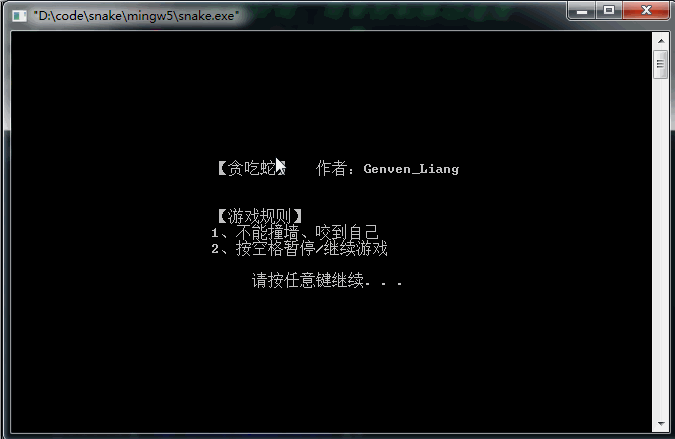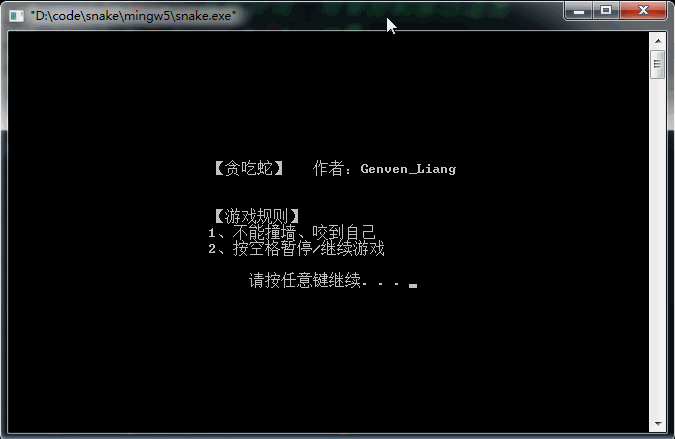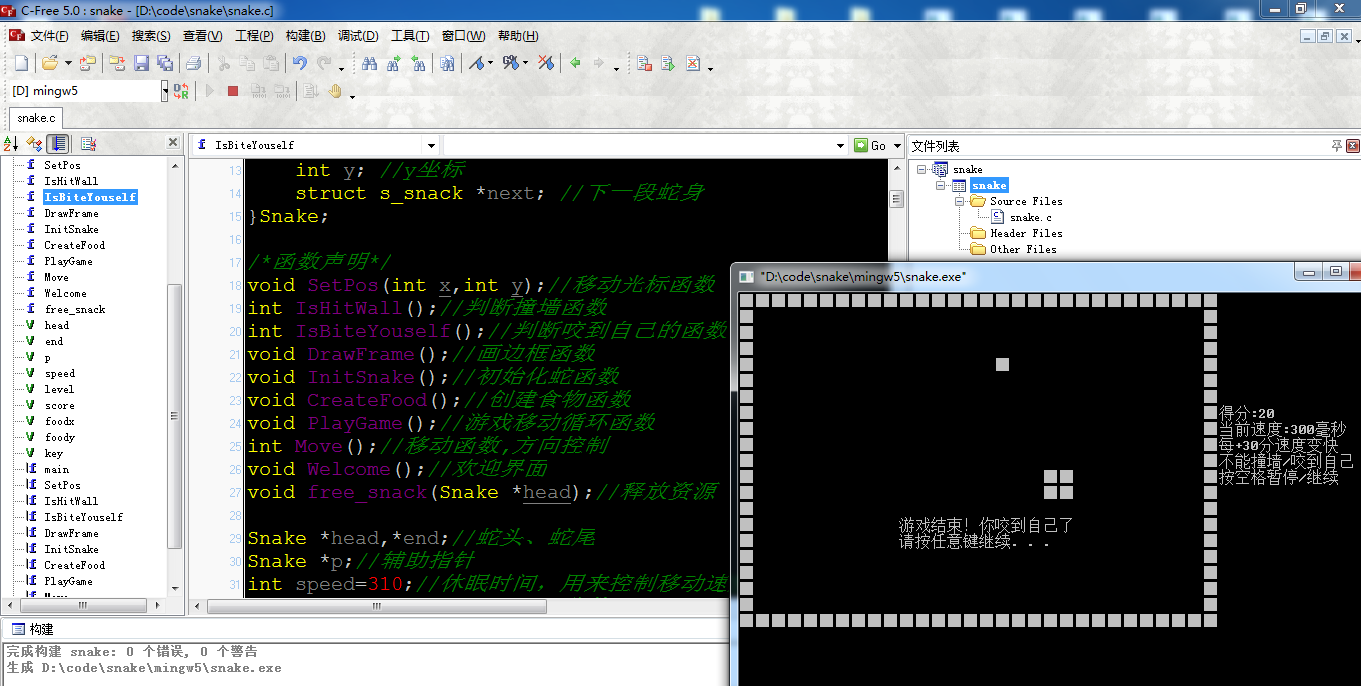#include <stdio.h>  //标准输入输出函数库
#include <stdlib.h> //包含system函数
#include <windows.h>//包含Sleep函数，来控制速度
#include <time.h>   //设置食物时随机生成坐标用到time做种子

#define DOWN_WALL 20	 //下边框  (下面的墙)   //使用宏定义 ，是方便以后调整边框大小
#define RIGHT_WALL 58 //右边框 (右面的墙)

//加上typedef 以后声明此类型的结构体不需要struct关键字，可以用这样声明 Snake s1;(原来：struct s_snake s1)
typedef struct s_snake //用来存储每一段蛇身的坐标位置
{
int x; //x坐标
int y; //y坐标
struct s_snake *next; //下一段蛇身
}Snake;

/*函数声明*/
void SetPos(int x,int y);//移动光标函数
int IsHitWall();//判断撞墙函数
int IsBiteYouself();//判断咬到自己的函数
void DrawFrame();//画边框函数
void InitSnake();//初始化蛇函数
void CreateFood();//创建食物函数
void PlayGame();//游戏移动循环函数
int Move();//移动函数,方向控制
void Welcome();//欢迎界面

Snake *p;//辅助指针
int speed=310;//休眠时间，用来控制移动速度
int level = 0,score = 0;//分数
int foodx,foody;//食物的（x，y）坐标
char key;//方向，暂停/继续 控制状态

int main()
{
Welcome(); //欢迎界面
DrawFrame();//画边框
InitSnake();//初始化蛇身
CreateFood();//创建食物

//右侧提示信息
SetPos(60,7);
printf("得分:%d",score);
SetPos(60,8);
printf("当前速度:%d毫秒",speed);
SetPos(60,9);
printf("每+30分速度变快");
SetPos(60,10);
printf("不能撞墙/咬到自己");
SetPos(60,11);
printf("按空格暂停/继续");

PlayGame();//按方向键控制蛇身进行游戏

return 0;
}

void SetPos(int x,int y)//设置光标位置(就是输出显示的开始位置)
{
/*  COORD是Windows API中定义的一种结构体
*	typedef struct _COORD
*	{
*		SHORT X;
*		SHORT Y;
*	} COORD;
*
*/
COORD pos = {x,y};
HANDLE output = GetStdHandle(STD_OUTPUT_HANDLE);//获得 标准输出的句柄
SetConsoleCursorPosition(output,pos); //设置控制台光标位置
}

int IsHitWall()//判断是否撞墙
{
{  //因为蛇头最先动，并且蛇身后一段下一步会在前一段，所以只要蛇头不撞墙，那么整个蛇身就不会 撞墙
SetPos(DOWN_WALL,14);
printf("游戏结束！撞到墙了\n");
SetPos(DOWN_WALL,15);//令 ‘按任意键继续...’居中显示
//system("pause");//暂停
return 1;
}
return 0;
}

int IsBiteYouself()//判断是否咬到自己
{
while(p->next!=NULL)
{
p=p->next;
{
SetPos(DOWN_WALL,14);
printf("游戏结束！你咬到自己了\n");
SetPos(DOWN_WALL,15);//令 “请按任意键继续”居中显示
//system("pause");//暂停
return 1;
}
}
return 0;
}

void DrawFrame()//画边框
{
int i = 0;
for(i=0;i<60;i+=2)//打印上下边框 注意i 一段蛇身占用 x 2个单位， y 1个单位
{
SetPos(i,0);   //上边框
printf("■");
SetPos(i,DOWN_WALL);//下边框
printf("■");
}
for(i=1;i<DOWN_WALL;i++)//打印左右边框
{
SetPos(0,i); //左边框
printf("■");
SetPos(RIGHT_WALL,i); //右边框
printf("■");
}
}

void InitSnake()//初始化蛇身  头插法	初始化从(20,15)开始的四段蛇身 (横向排列)
{
int i = 0;

//创建一个蛇身位置 蛇尾
end=(Snake*)malloc(sizeof(Snake));
end->x=20;
end->y=15;
end->next=NULL;

//创建三个蛇身位置
for (i=1;i<=3;i++)
{
head->x = 20+2*i; //每个蛇身 x相差 2个单位

}

//从蛇头开始画贪吃蛇
while (end->next!=NULL)
{
SetPos(end->x,end->y);
printf("■");
end = end->next;
}
}

void CreateFood()//设置食物
{
srand(time(0));//设置随机数种子
flag:
while(1)//由于food的x坐标必须为偶数，所以设置循环判断是否为偶数
{
//rand()%num产生 0~num-1
//rand产生范围数公式rand()%(m+1-n)+n;有效范围在 [n,m]
foody=rand()%(DOWN_WALL-1+1-1)+1;//foody的有效范围在 [1,DOWN_WALL-1 ]
foodx=rand()%(RIGHT_WALL-2+1-3)+3;//foodx的有效范围在    [3,RIGHT_WALL-2] 注意x是以2为单位的
if (foodx%2==0)
{
break;
}
}
while(1)
{
if(p->x==foodx&&p->y==foody)//若生成坐标和蛇重叠了，回到生成坐标循环
{
goto flag;
}

if(p->next==NULL) //与每一段蛇身比较完毕，跳出循环
{
break;
}
p=p->next;
}

SetPos(foodx,foody);
printf("■");  //显示食物
}

void PlayGame()//贪吃蛇移动，暂停
{
int mv_ret = 0;//移动后的返回值，如果撞墙、或就咬到自己设置为1
key = 'd';//刚开始，贪吃蛇默认向右移动
while (1)
{
//GetAsyncKeyState(VK_UP) 判断VK_UP按键的状态，若是被按下，则位15设为1；如抬起，则为0
//所以要 与上0x8000 取出第15位 进行判断
if ((GetAsyncKeyState(VK_UP)&& 0x8000)&& key != 's')//与key!='s'，因为不能后退
{
key='w';//如果本来不是向下的，按下向上键，将key设置为w
}
else if ((GetAsyncKeyState(VK_DOWN)&& 0x8000)&& key != 'w')
{
key='s';
}
else if ((GetAsyncKeyState(VK_RIGHT) && 0x8000)&& key != 'a')
{
key='d';
}
else if ((GetAsyncKeyState(VK_LEFT)&& 0x8000) && key != 'd')
{
key='a';
}
else if (GetAsyncKeyState(VK_SPACE)&& 0x8000) //暂停/继续函数
{
//补上消隐的蛇尾(蛇尾还在)  原因未知
while(p->next!=NULL) p=p->next;
SetPos(p->x,p->y);
printf("■");

while(1)//暂停语句
{
Sleep(100); //必要延时(消抖) Sleep(毫秒)
if (GetAsyncKeyState(VK_SPACE)&& 0x8000)
{
break;
}
}

//擦掉补上的 蛇尾
SetPos(p->x,p->y);
printf(" ");
}
else if(GetAsyncKeyState(VK_ESCAPE)&& 0x8000)//按下ESC退出游戏，VK_ESCAPE == 27
{
return ;
}

//实时刷新速度  得分每+30分 移动速度变快
if (score==level && speed > 10)//判断得分
{
speed -=10; //睡眠时间，改变移动速度，越少越快
level +=30; //速度变快条件 变化

SetPos(60,8);
printf("当前速度:%d毫秒",speed);
}
mv_ret = Move();//移动蛇身
if( mv_ret == 1)
{
break;
}
}
}

int Move()//移动函数，w前 s后 a左 d右，实现移动:头部增加一个，尾部减掉一个
{
int ret;

//不是按下控制方向的 a,s,d,w 就
if( (key != 'a') && (key != 's') && (key != 'd') && (key != 'w') )
{
return 0;
}

ret = IsHitWall(); //是否撞墙
ret += IsBiteYouself(); //是否咬到自己
if(ret == 1)
{
return 1;
}

p = (Snake*)malloc(sizeof(Snake));//头部增加的那个

switch(key)
{
case 'd'://向右
break;

case 'w'://向上
break;

case 's'://向下
break;

case 'a'://向左
break;

}

//画出新的头部
SetPos(p->x,p->y);
printf("■");

//如果 移动的一格刚好是食物的位置,新增的称为蛇头，不用去掉蛇尾
//如果 移动的一格不是食物的位置，新增的称为蛇头，去掉蛇尾，就是贪吃蛇移动一格的效果

Sleep(speed);//移动速度的控制
if (p->x==foodx && p->y==foody)//移动的一格刚好是食物的位置
{
CreateFood();
score +=10;
SetPos(60,7);
printf("得分:%d",score);

}
else   //吃不到食物，头部添加一个，尾部去掉一个
{
//移动的一格刚好是食物的位置,新增的称为蛇头，不用去掉蛇尾
while (p->next->next != NULL) p = p->next;//指向蛇尾前一格，然后释放蛇尾，并将原来的尾二置空。

SetPos(p->x, p->y);//为什么不是setPos(p->next->x,p->next->y)?
printf(" ");//擦掉蛇尾(蛇头加一，蛇尾减一，实现移动效果)

free(p->next);//释放蛇尾
p->next = NULL;//必须置空，不然原来的尾二还是指向原来的尾巴(但是原来的为尾巴已经释放了)
}

return 0;
}

void Welcome()//欢迎界面
{
SetPos(25,8);
printf("【贪吃蛇】   作者：Genven_Liang");
SetPos(25,11);
printf("【游戏规则】");
SetPos(25,12);
printf("1、不能撞墙、咬到自己");
SetPos(25,13);
printf("2、按空格暂停/继续游戏");

printf("\n");
SetPos(30,15);
system("pause");//暂停
system("cls");//清屏
}

{
{
return ;
}

//从头部开始逐个节点释放
{
free(p);
}
}


例子使用的是C-Free，这个IDE在程序结束后会暂停的；如果使用的是Visual Studio，程序结束后不会暂停；需要在IsHitWall()函数和IsBiteYouself()函数里面加上一句system(pause);暂停一下(或者是加上一句getchar())，这样就能看见"游戏结束"了。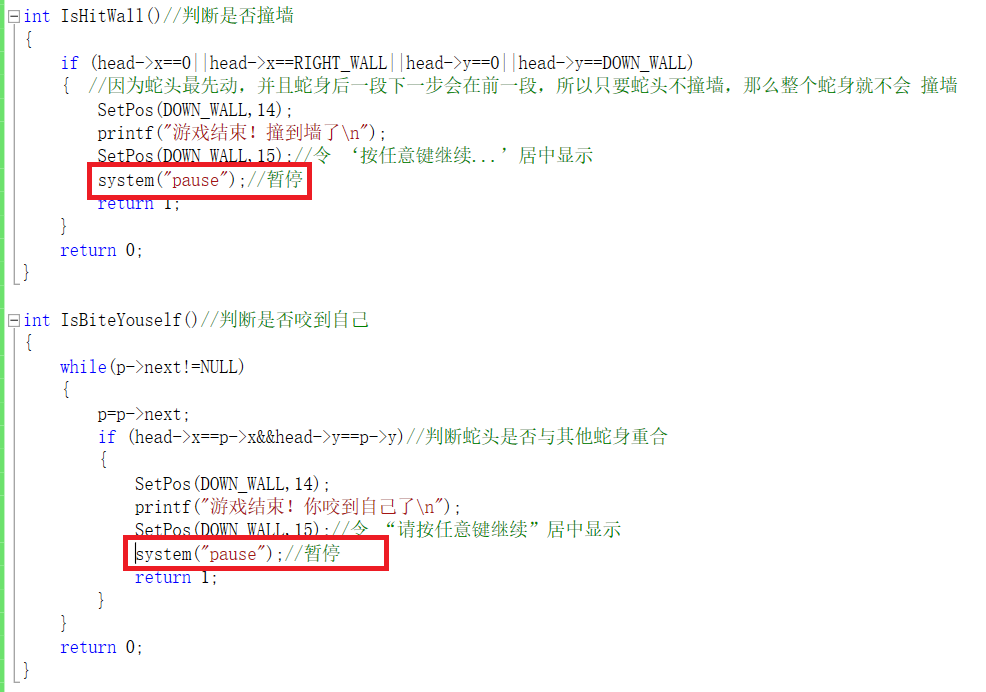6.1 残留问题

目前在Win10-64bit家庭中文版 发现残留问题，现象如下：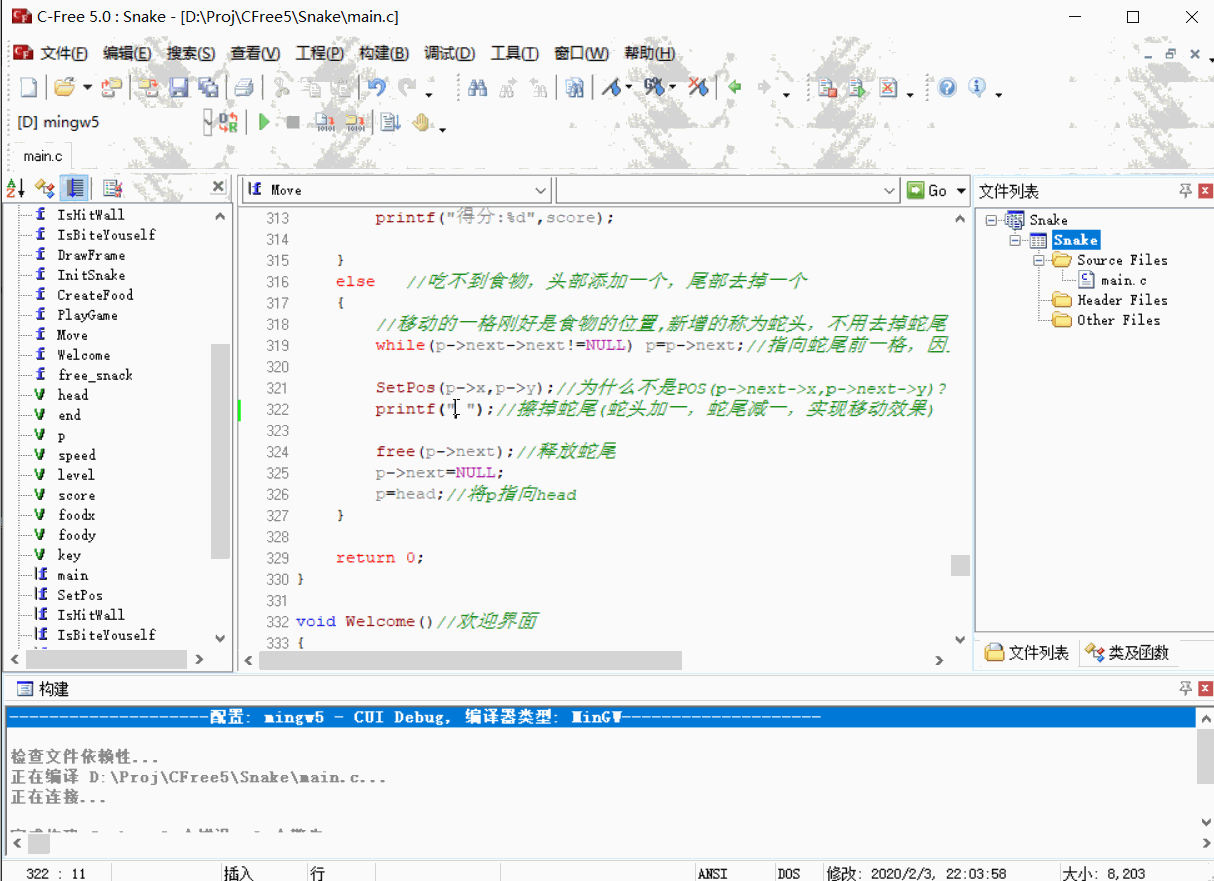ps:在Win7-32bit 系统，Win7-64bit系统，Win10专业版未发现该问题。

产生原因:空格的宽度与■的宽度不一致，导致用空格不能完全擦除一段完整的蛇身--■

解决办法:使用两个空格来擦除一段蛇身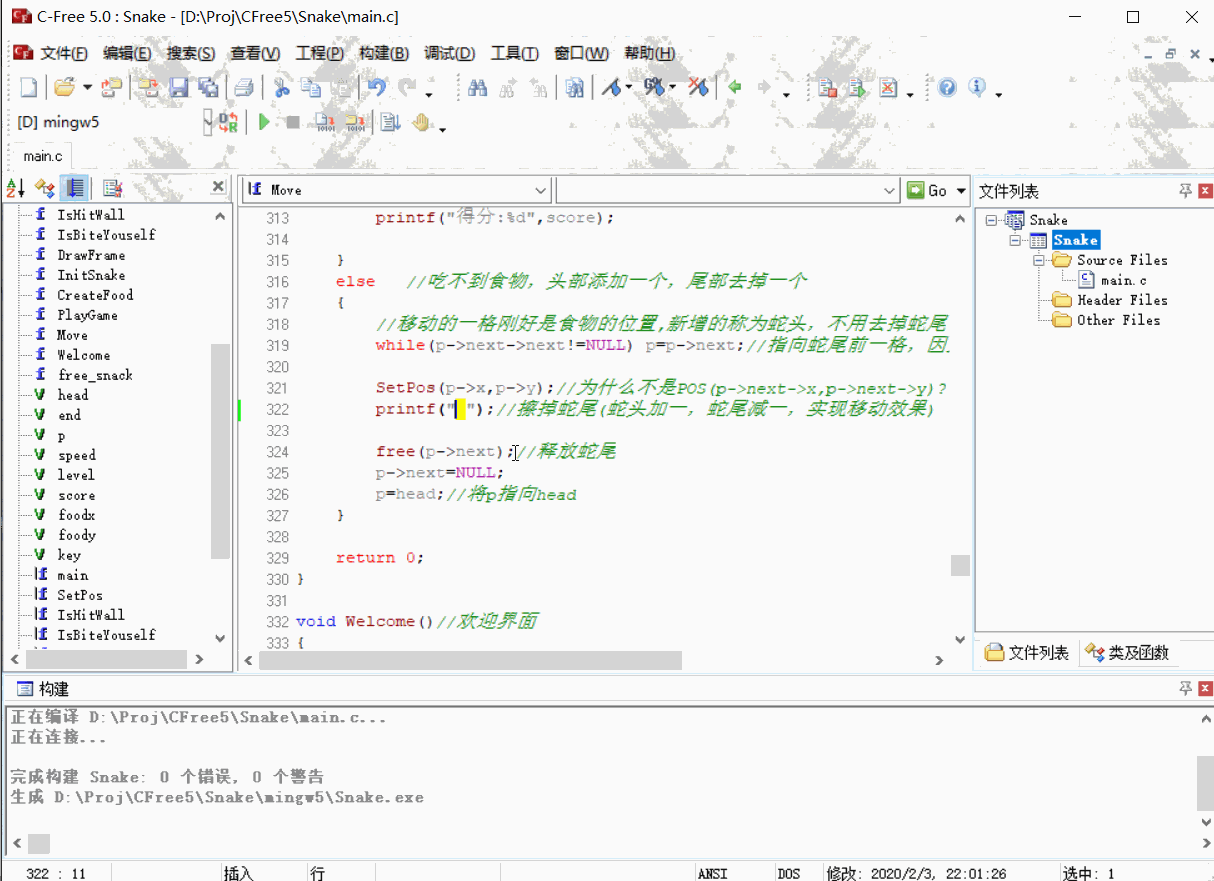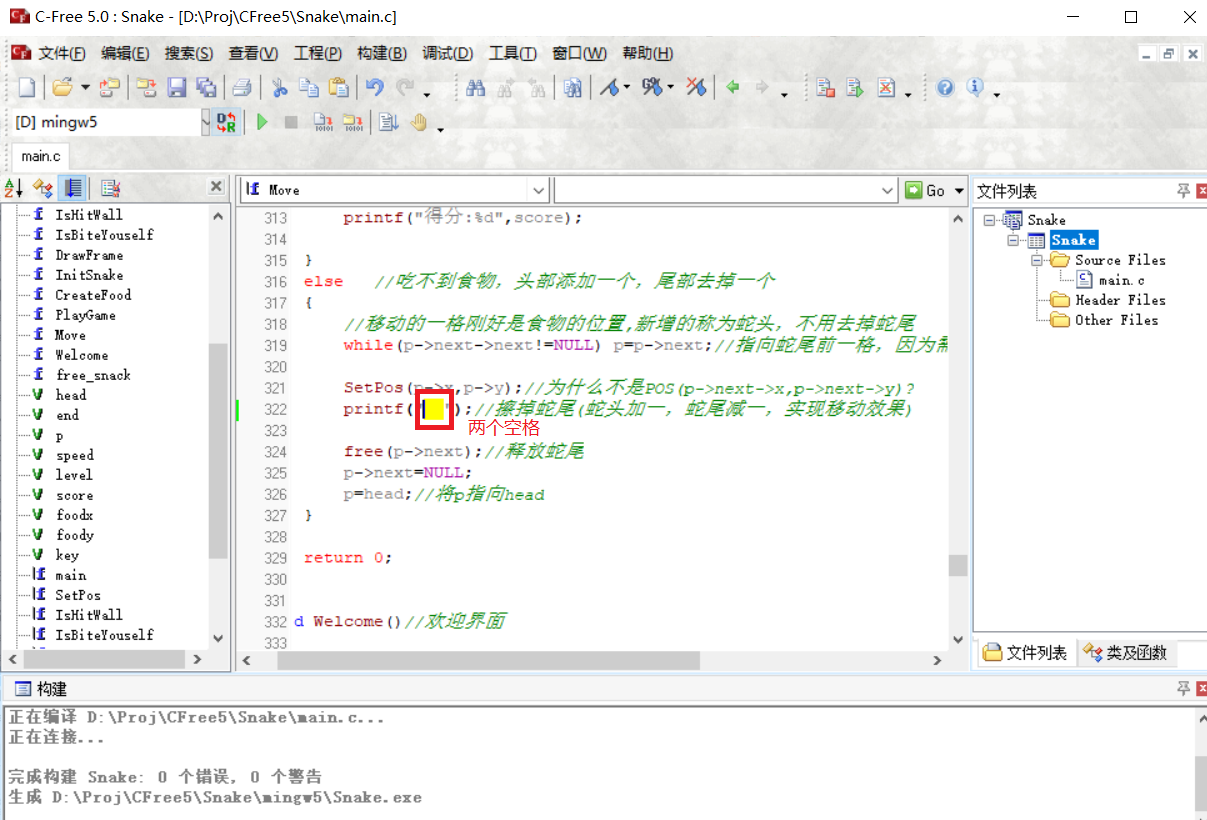===========================以下回复 qq_45936030 这位老铁===================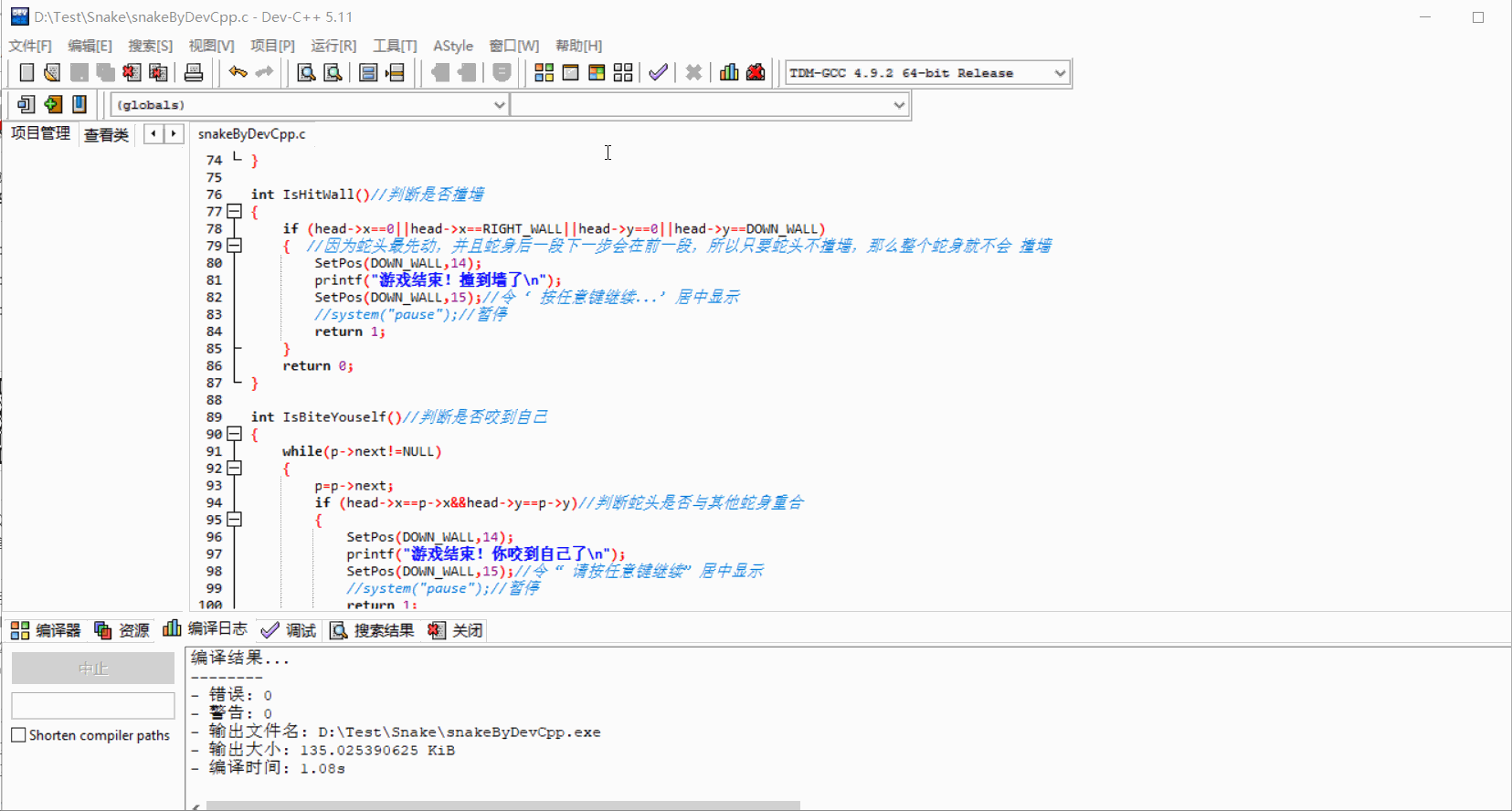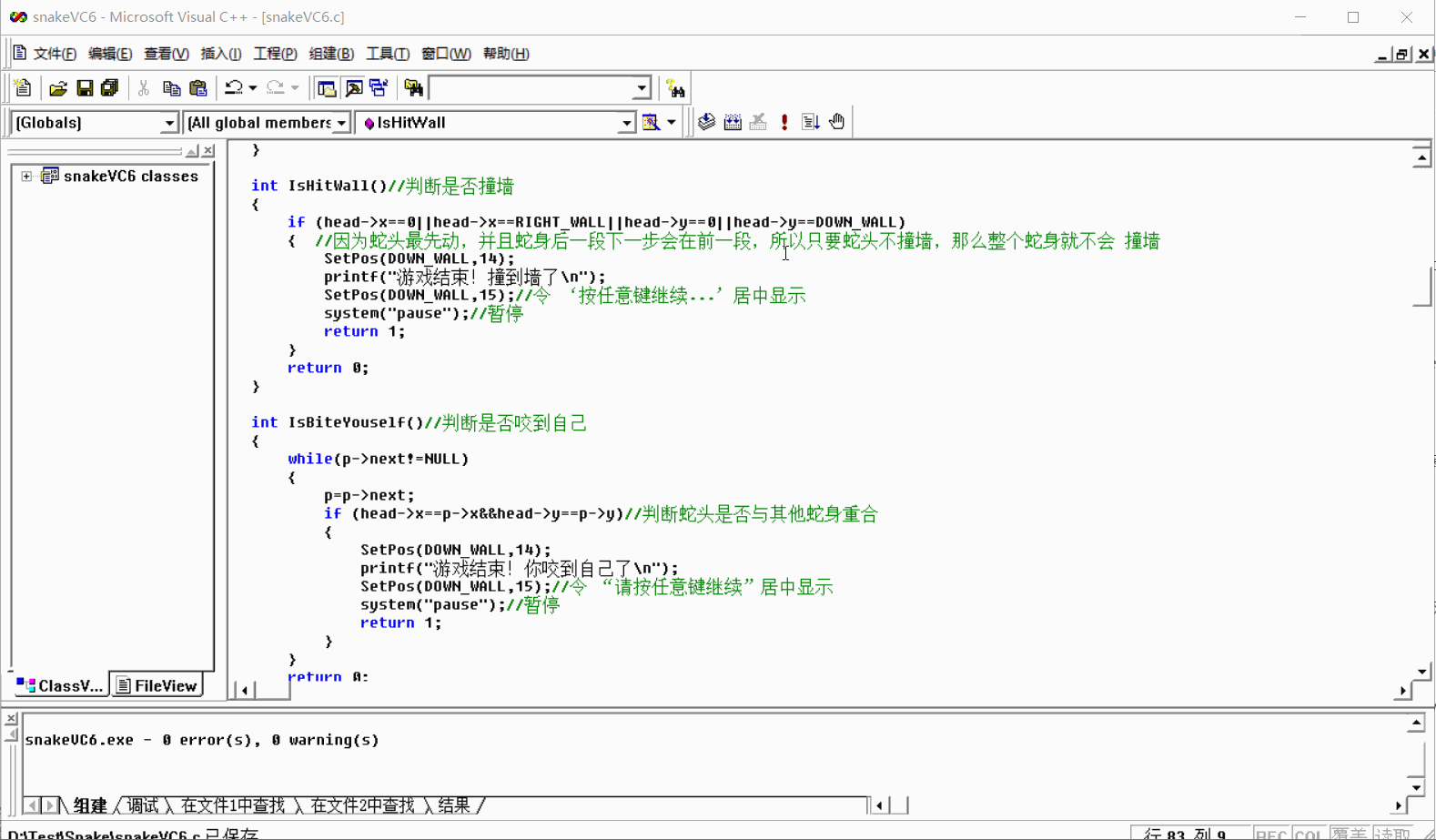====================================以下回复  钢钢钢不爽 这位老铁 ======================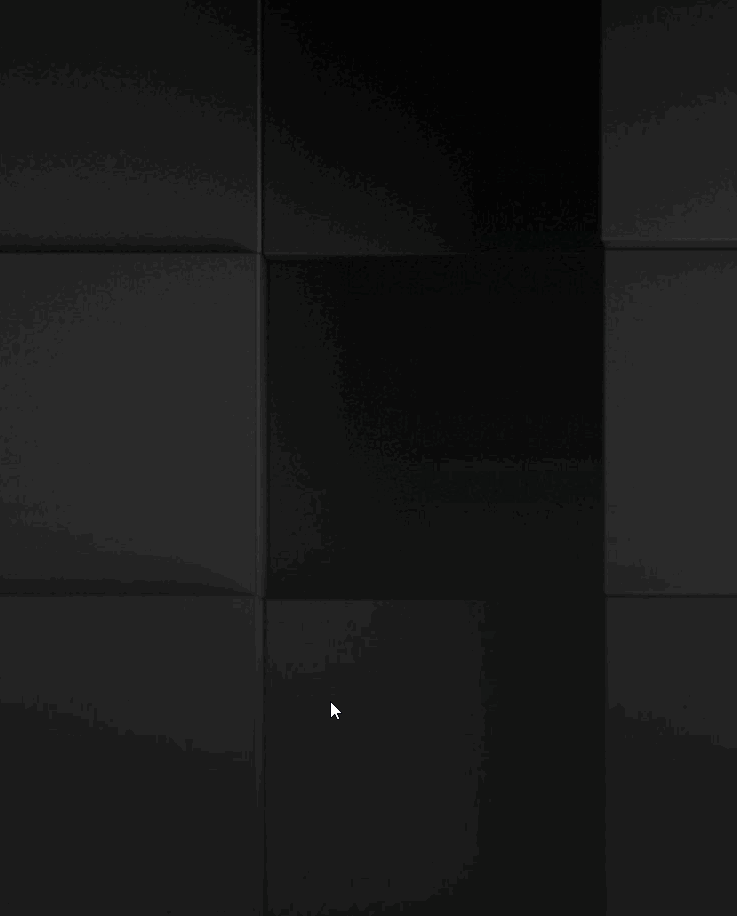ps:还可以使用系统自带的造字程序进行自定义，搜索:专用字符编辑程序 或在运行窗口直接输入 eudcedit.exe 然后回车。

======================以下回复  xmx666 这位老铁 ===============

1 p->next->next是对的，p->next是不对的。以下是p->next->next和p->next的对比：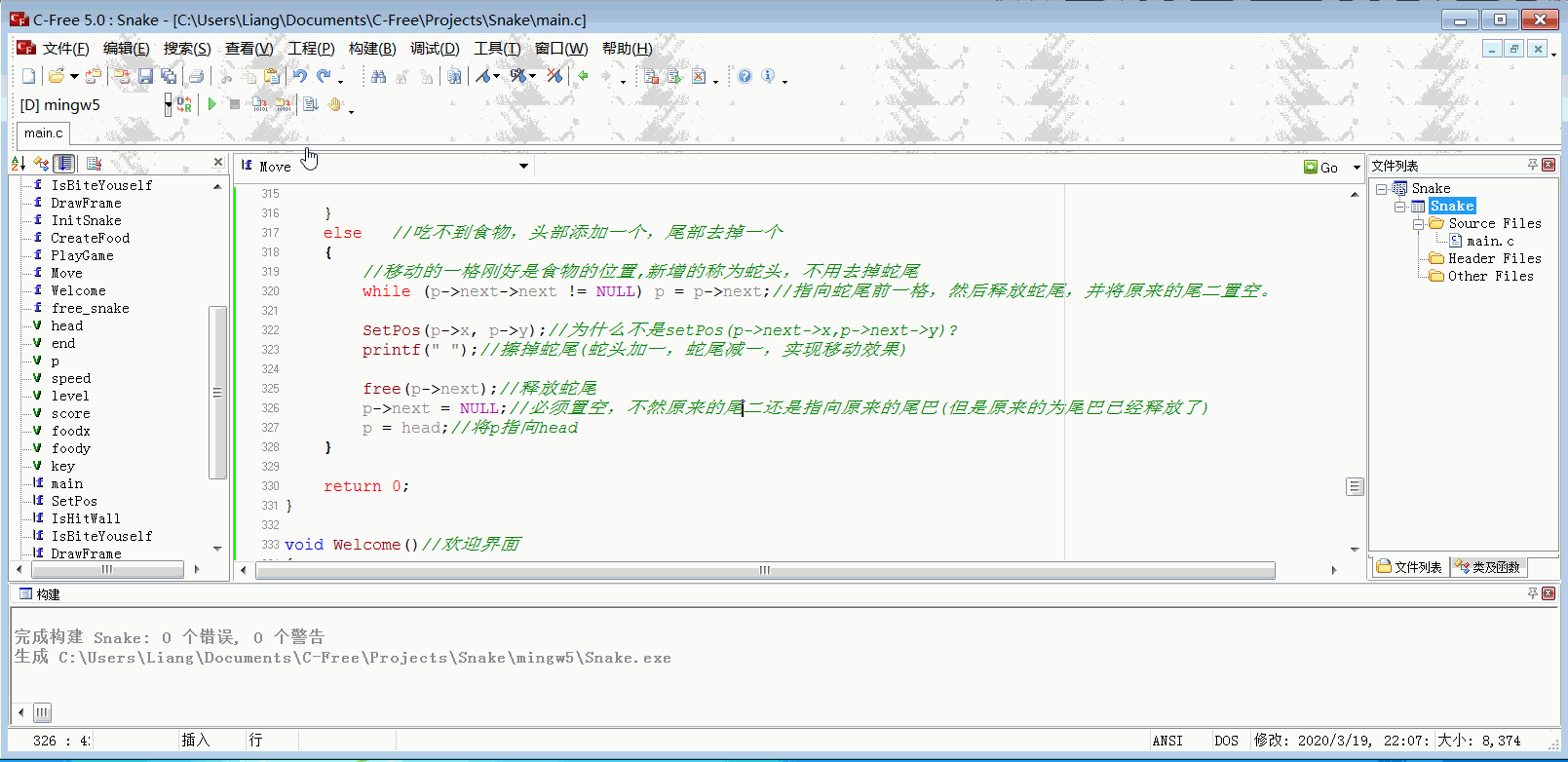去掉最后一个方块有2个操作：1）将最后的方块A释放内存，擦除方块A；2）断开联系，方块A的前面的方块B不要再指向方块A。

（如果不断开的话，B还是指向A，但是A已经被释放了，程序已经没有合法权限去的操作A的内存空间了。）

其中while (p->next->next != NULL) p = p->next;是找到倒数第二个方块，

释放方块A的空间不管是找到A还是A的前一个都是可以的，但是单项链表不能操作A的前一个。

将A的前面一个的next置空，如果是使用p->next找到A并释放A，那么是不能直接操作A的前面一个的(但是可以增加一个变量用来指向A的前面一个)

07-02
05-263万+点击重新获取扫码支付余额充值